Worksheets and No Prep Teaching Resources
Math Worksheets
Measurement Worksheets

# WeightWorksheets, Lessons, and Printables

Length (black and white graphics)
Circle the object with the longest side
Number the objects in order from shortest to longest
Number the objects in order from longest to shortest
Estimate (inches and feet)
Estimate (centimeters and meters)
Weight (black and white graphics)
Circle the object that is heavier
Circle the object that is lighter
Number the objects in order from lightest to heaviest
Number the objects in order from heaviest to lightest
Estimate (ounces and pounds)
Estimate (grams and kilograms)
Review (black and white graphics)
Length and weight review (black and white graphics)

Length (color graphics)
Circle the object with the longest side
Number the objects in order from shortest to longest
Number the objects in order from longest to shortest
Estimate (inches and feet)
Estimate (centimeters and meters)
Weight (color graphics)
Circle the object that is heavier
Circle the object that is lighter
Number the objects in order from lightest to heaviest
Number the objects in order from heaviest to lightest
Estimate (ounces and pounds)
Estimate (grams and kilograms)
Review (color graphics)
Length and weight review (color graphics)

Make the Scale Balance
Easier: Balance is Equal - Add the Missing Blocks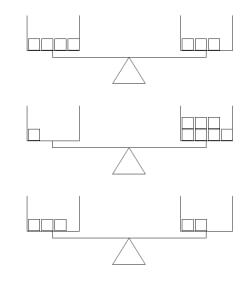Add the missing blocks Add the missing blocks and write the number of blocks on each side Add the missing blocks - one problem does not need any blocks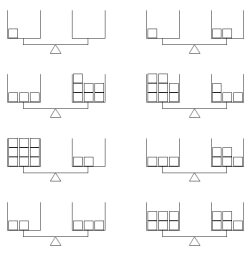More problems per page More problems per page - one problem does not need any blocks

One Side of Balance is Heavier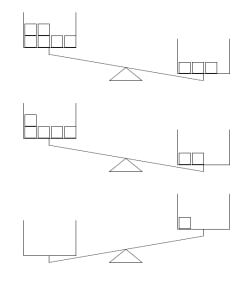Add the missing blocks Add the missing blocks and write the number of blocks on each side Add the missing blocks - one problem does not need any blocks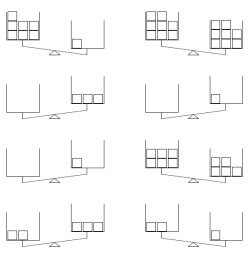More problems per page More problems per page - one problem does not need any blocks

Some Balances are Equal - Others have Heavier Sides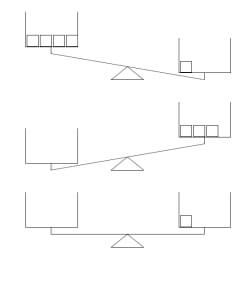Add the missing blocks Add the missing blocks and write the number of blocks on each side Add the missing blocks - one problem does not need any blocks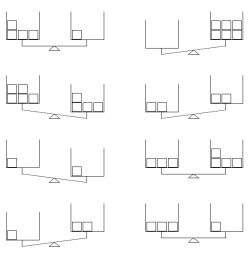More problems per page More problems per page - one problem does not need any blocks

Greater and Less Than: Make the Scale Balance
Easier: Maximum Sum of 19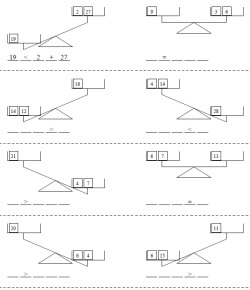Answer given for the first problem - the sign is given for all the problems Answer given for the first problem - the sign is given for the first four problems Answer given for the first problem No answers, but blank lines are shown for parts ___ ____ ____ ____ _____
Maximum Sum of 99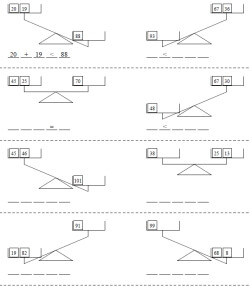Answer given for the first problem - the sign is given for all the problems Answer given for the first problem - the sign is given for the first four problems Answer given for the first problem No answers, but blank lines are shown for parts ___ ____ ____ ____ _____
Algebra Challenge - Maximum Sum of 19 - All balances are equal and there is one UNKNOWN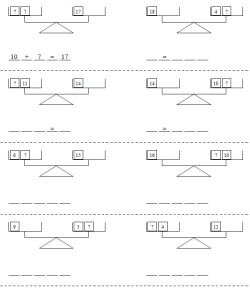Algebra Challenge: Answer given for the first problem - the sign is given for all the problems Algebra Challenge: Answer given for the first problem - the sign is given for the first four problems Algebra Challenge: Answer given for the first problem Algebra Challenge: No answers, but blank lines are shown for parts ___ ____ ____ ____ _____

Measurement Logic Puzzles
Mass, volume, and density
Mass, volume, and density (with decimals)
Shipping packages

Measurement
Metric units of mass

English Measurement
English Weight

Metric System: Problem Solving Puzzles
Measuring Weight: Shipping Packages (Grams and Kilograms)

Metric System: Metric System Worksheets
Metric Weight

Metric System: Metric System Crossword Number Puzzles
Metric Weight

Measurement

Have a suggestion or would like to leave feedback?